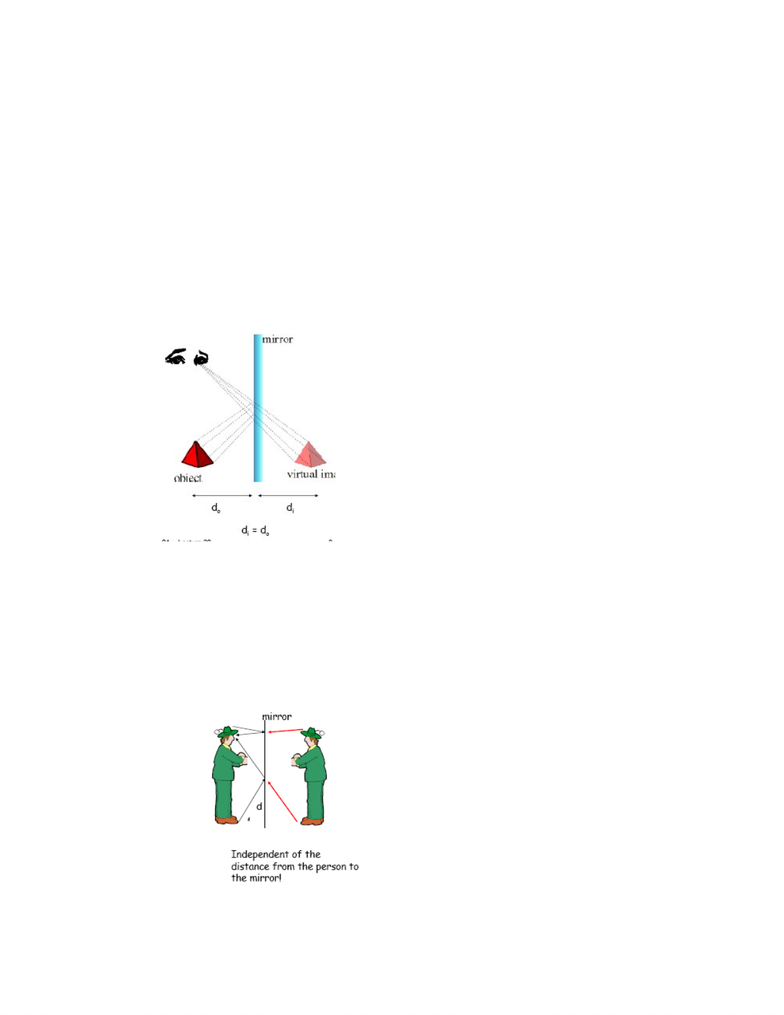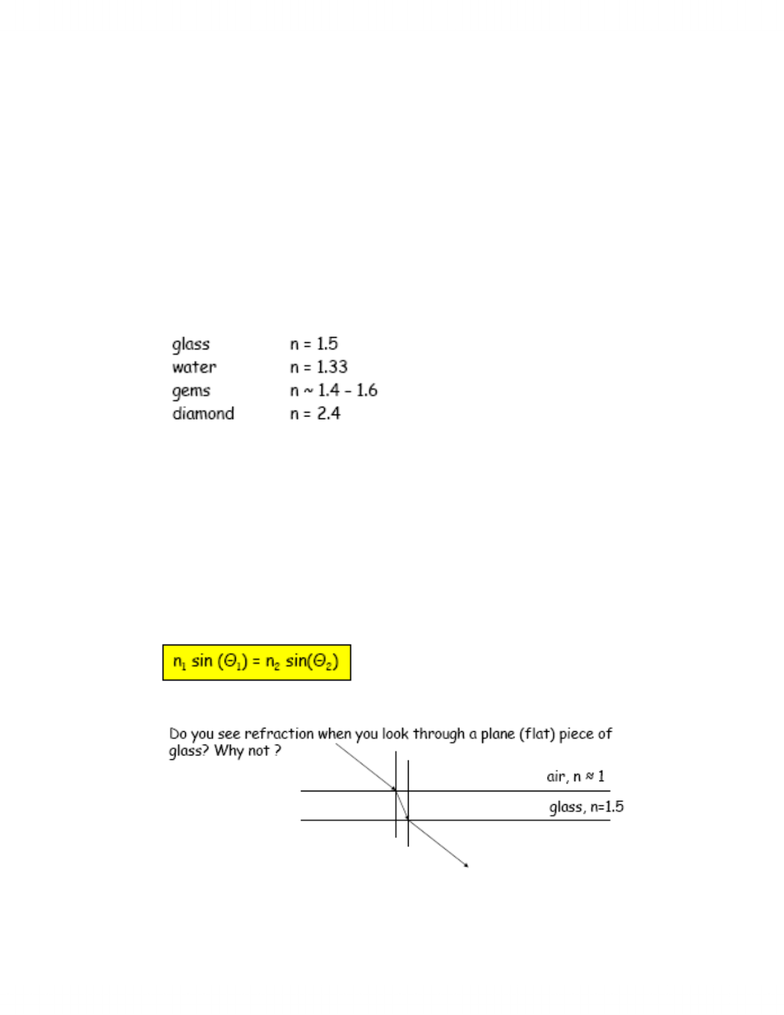Class Notes (1,100,000)
CA (620,000)
McGill (30,000)
Physics (500)
Lecture

# PHYS 101 Lecture Notes - Real Image, Refraction, Total Internal Reflection

Department
Physics
Course Code
PHYS 101
Professor
Kenneth Ragan

This preview shows pages 1-2. to view the full 7 pages of the document.Phys 101 Alanna Houston
November 15, 2007
Light Propagation:
-Light usually travels in straight lines (sharpness of shadows,
beams such as headlights, laser pointers etc.)
-Therefore, we usually use the ray model of light
oDescribes reflection, refraction, and behaviour of light in
lenses and mirrors
oThis is called geometrical optics
Reflection:
-Angle of incidence = angle of reflection
-Angles measures from the normal to the surface
-In a plane (flat) mirror:
-
olight rays emitted from object or reflected from object, strike
the mirror, and are reflected into the observer’s eyes. She
extrapolates these rays backwards and perceives an image
“behind the mirror”
oimage called a virtual image and appears at the image
distance di behind the mirror
oHere the image distance di is equal to the object distance do
-Example: Minimum height of mirror necessary for 1.60m person to
see himself? Assume eyes are 10 cm below the top of the head.
o
ouse 5 cm down because the angles are the same

Only pages 1-2 are available for preview. Some parts have been intentionally blurred.Phys 101 Alanna Houston
othetai = thetar
o1.60-0.10 = 1.50 cm
otherefore, from the feet to the mirror would be 75 cm
ox=160 cm – 75 cm – 5 cm therefore x = 80 cm
Refraction:
-the ration of speed of light in a vacuum (written as c) to the speed
of light in a medium is called the index of refraction: n = c/vlight. C
= 3.00x10^8 m/s (speed of light in a vacuum) Therefore, the
index of refraction in a vacuum is 1.0
-vlight<c for all materials: n for all materials is larger than 1.0
-occurs when light is incident on a boundary where the index of
refraction changes.
-Typical indices of refraction:
-
-index of refraction is often a slight function of the wavelength
(colour) of the light
-For example, for some prism glass, n(blue) = 1.525 and n(red) =
1.514
-The large index of refraction and the large variation of n with l
(dispersion) are reasons that diamonds sparkle…the large index of
refraction makes total internal reflection more probably and the
number of bounces inside the diamond larger
-At each bounce, the difference in the colours is enhanced so the
diamond ‘flashes’ with brilliance and colour
-In general, there will be part of the ray that reflects and part of the
ray that refracts:
- (Snell’s Law)
-If n2 is greater than n1, the ray bends towards the normal (less
dense to more dense)
-
-Example: What is the apparent depth of a pool of real depth 1.0m?
###### You're Reading a Preview

Unlock to view full version